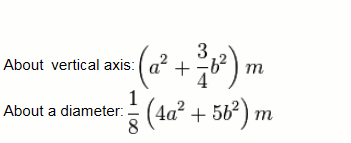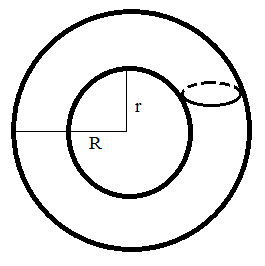# Moment of Inertia of a torus Calculator

This CalcTown Calculator calculates the Moment of Inertia of a torus, both along its diameter and the vertical axis.

Kg
m
m

#### Result

Kg-m2
Kg-m2Click here to view image

where

a=tube radius of the torus

b=cross sectional radius of the torus

m=mass of the torus